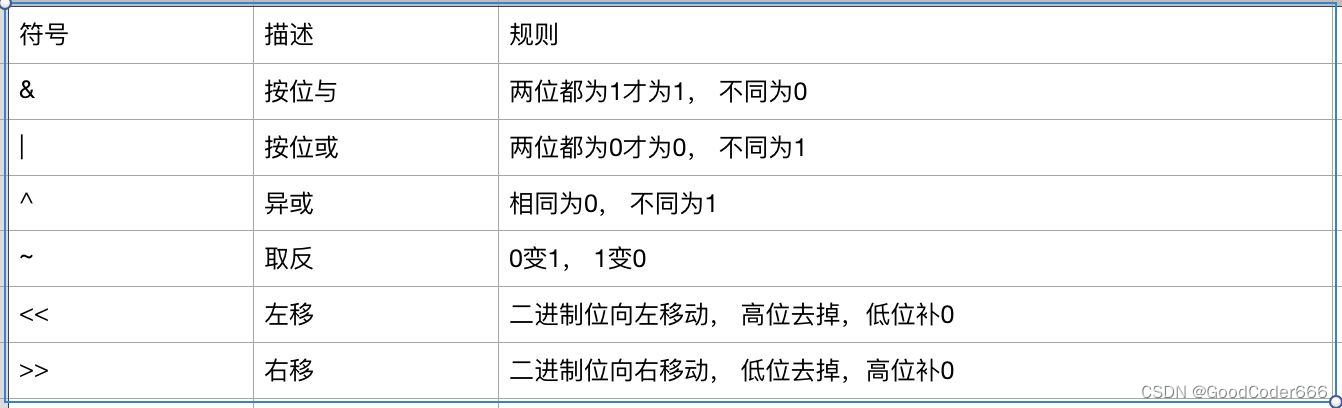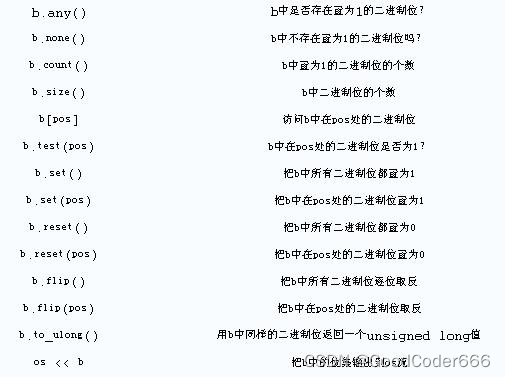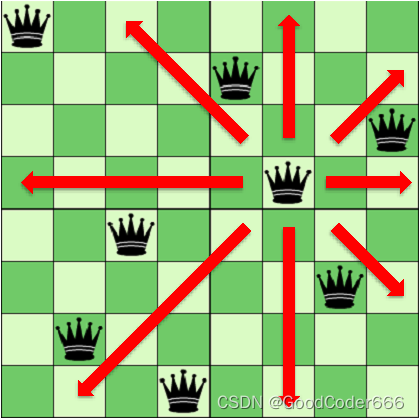# 【算法笔记】位运算详解

## 1. 基本运算### 1.1 取反

~0011 = 1100
~1011 = 0100

### 1.2 按位与

x x y y x & y
0 0 0 0 0 0
0 0 1 1 0 0
1 1 0 0 0 0
1 1 1 1 1 1

0011 & 1100 = 0000
1010 & 1011 = 1010

• 交换律：a & b = b & a
• 结合律：a & b & c = a & (b & c)
• 自与：a & a = a
• 0 0 0 & a1 & a2 & a3 & ... = 0
• ∞ \infty （全 1 1 ）：a & inf = a

### 1.3 按位或

x x y y x | y
0 0 0 0 0 0
0 0 1 1 1 1
1 1 0 0 1 1
1 1 1 1 1 1

1100 | 0011 = 1111
1010 | 0001 = 1011

• 交换律：a | b = b | a
• 结合律：a | b | c = a | (b | c)
• 自或：a | a = a
• 0 0 a | 0 = a
• ∞ \infty （全 1 1 ）：a | inf = inf

### 1.4 异或

x x y y x ⊕ y x\oplus y
0 0 0 0 0 0
0 0 1 1 1 1
1 1 0 0 1 1
1 1 1 1 0 0

1000 ^ 1011 = 0011
0101 ^ 1010 = 1111

• 交换律： a ⊕ b = b ⊕ a a\oplus b=b\oplus a
• 结合律： a ⊕ b ⊕ c = a ⊕ ( b ⊕ c ) a\oplus b\oplus c=a\oplus(b\oplus c)
• 自异或： a ⊕ a = 0 a\oplus a=0
• 异或 0 0 a ⊕ 0 = a a\oplus 0=a
• 多重异或： a ⊕ b ⊕ b = a ⊕ ( b ⊕ b ) = a ⊕ 0 = a a\oplus b\oplus b=a\oplus (b\oplus b)=a\oplus 0=a
• 异或 ∞ \infty （全 1 1 ）： a ⊕ ∞ =   a\oplus \infty=~ ~a
• a ⊕ b = c a\oplus b=c ，则 a ⊕ c = b a\oplus c=b

### 1.5 位移

• a << b：将 a a 末尾添上 b b 0 0 的结果。
• a >> b：从 a a 末尾删掉 b b 位的结果。

• (a << b) >> b = a
• a << b   = a × 2 b ~=a\times 2^b
• a >> b   = ⌊ a 2 b ⌋ ~=\lfloor\frac a {2^b}\rfloor

### 1.6 练习题

#### 1.6.2 洛谷 P1100 高低位交换

x x A A B B
x >> 16 16 16 0 0 A A
x << 16 B B 16 16 0 0
ans B B A A

#include <cstdio>
using namespace std;

int main()
{
unsigned int x;
scanf("%u", &x);
printf("%u\n", (x >> 16) | (x << 16));
return 0;
}


#### 1.6.3 找出不同的数

- 时间 O ( N ) \mathcal O(N) O ( N log ⁡ N ) \mathcal O(N\log N) ，空间 O ( N ) \mathcal O(N) 解法

- 时间 O ( N ) \mathcal O(N) ，空间 O ( 1 ) \mathcal O(1) 解法

#include <cstdio>
using namespace std;

int main()
{
int n;
scanf("%d", &n);
n = (n << 1) + 1;
int ans = 0;
while(n--)
{
int x;
scanf("%d", &x);
ans ^= x;
}
printf("%d\n", ans);
return 0;
}


#### 1.6.4 AtCoder Beginner Contest 261 E - Many Operations

// https://atcoder.jp/contests/abc261/submissions/33495431
#include <cstdio>
using namespace std;

int main()
{
unsigned n, c, zero = 0, one = 0xffffffff;
scanf("%d%d", &n, &c);
while(n--)
{
int t, a;
scanf("%d%d", &t, &a);
if(t == 1) one &= a, zero &= a;
else if(t == 2) one |= a, zero |= a;
else one ^= a, zero ^= a;
printf("%d\n", c = (c & one) | (~c & zero));
}
return 0;
}


## 2. 扩展概念&运算

### 2.1 lowbit

lowbit(x)即为二进制下 x x 的最低位，如lowbit(10010) = 10lowbit(1) = 1。严格来说 0 0 没有lowbit，部分情况下可视为lowbit(0) = 1。利用lowbit函数可实现树状数组等数据结构。

lowbit 计算方式

1. 暴力计算
简单粗暴的按位直接计算，如下：
int lowbit(int x)
{
int res = 1;
while(x && !(x & 1))
x >>= 1, res <<= 1;
return res;
}

时间复杂度 O ( log ⁡ X ) \mathcal O(\log X) 。缺点：速度慢，代码长，没有体现位运算的优势
2. x & -x
巧妙利用lowbit(x) = x & -x。感兴趣的读者可自行尝试证明。
时间复杂度 O ( 1 ) \mathcal O(1) 。相比(1)来说，代码更短，速度更快。
3. x & (x - 1)
注意：x & (x - 1)不是lowbit(x)，而是x - lowbit(x)
这种方法常用于树状数组中，可提升x - lowbit(x)的计算速度。

### 2.2 popcount

popcount(x)定义为 x x 在二进制下 1 1 的个数，如popcount(10101) = 3popcount(0) = 0

popcount 计算方式

1. 暴力计算检查
还是最粗暴的算法，通过枚举每一位并检查是否为 1 1 达到目的，时间复杂度为 O ( log ⁡ X ) \mathcal O(\log X)
int popcount(int x)
{
int res = 0;
while(x)
{
res += x & 1;
x >>= 1;
}
return res;
}

2. lowbit 优化
时间复杂度还是 O ( log ⁡ X ) \mathcal O(\log X) ，不过平均用时会比(1)快2~3倍左右。
int popcount(int x)
{
int res = 0;
for(; x; x&=x-1) res ++;
return res;
}

3. builtin 函数（最快）
详见3.1 __builtin_popcount/__builtin_popcountll

## 3. builtin 位运算函数

### 3.5 __builtin_parity / __builtin_parityll

P.S. 这函数，不知是哪位神仙想出来的……

## 4. 位运算的应用

### 4.1 子集表示法

#### 4.1.1 子集操作

• 空集： 0 0
• 满集： 2 N − 1 2^N-1 N N 1 1
• 集合 S S 的元素个数：__builtin_popcount(S)__builtin_popcountll(S)
• 集合 S S 是否包含 i i S >> i & 1
• i i 加入 S S （操作前 S S 是否包含 i i 不影响操作结果）：S |= 1 << i
• i i S S 中删除（操作前 S S 必须包含 i i ）：S ^= 1 << i
• i i S S 中删除（操作前 S S 是否包含 i i 不影响操作结果）：S &= ~(1 << i)
• S S T T 的交集（ S S T T 都包含的集合）：S & T
• S S T T 的并集（ S S T T 中有任意一个包含的集合）：S | T
• S S T T 的差集（ S S T T 中恰好有一个包含的集合）：S ^ T

#### 4.1.2 子集枚举

- 必会：枚举 N N 个元素的所有子集

#include <cstdio>
using namespace std;

const int N = 3;

int main()
{
printf("N = %d\n", N);
for(int s=0, full=(1<<N)-1; s<=full; s++)
{
printf("Subset %d:", s + 1);
for(int i=0; i<N; i++)
if(s >> i & 1)
printf(" %d", i);
putchar('\n');
}
return 0;
}


- 必会：枚举子集的子集

for(int S=0; S<(1<<N); S++) // 枚举子集S
for(int T=S; T; T=(T-1)&S) // 枚举子集的子集T
{
// Do something...
printf("%d\n", t);
}


- 扩展：枚举 N N 个元素中大小为 K K 的子集

for(int s=0; s<(1<<n); s++)
{
int cnt = __builtin_popcount(s);
if(cnt != K) continue;
// Do something...
}


int S = (1 << k) - 1;
while(S < 1 << n)
{
// Do something...
printf("%d\n", S);
// 移到下一个合法子集
int x = S & -S, y = S + x;
S = ((S & ~y) / x >> 1) | y;
}


#### 4.1.3 扩展：std::bitset

bitset，顾名思义，即为用位运算操作的集合。

C++的Standard Template LibrarySTL）为我们提供了<bitset>头文件，用于bitset的定义。#include <cstdio>
#include <bitset> // 头文件
using namespace std;

int main()
{
const int N = 500;
bitset<N> S; // 定义大小为N的bitset S，初始为全0
S.set(1);    // 将S的第1位设为1
S = 1;    // 将S的第0位设为1，注意bitset可使用下标访问和赋值
S.reset(1);  // 将S的第1位设为0
printf("S: %d\n", (int)S); // 输出S第2位上的值
printf("Count: %d\n", (int)S.count()); // S的popcount（二进制下1的个数）
printf("Size: %d\n", (int)S.size()); // S的二进制位数（N）
printf("None? %d\n", (int)S.none()); // S是否为空？
printf("Any?  %d\n", (int)S.any());  // S是否有1？
bitset<N> T; // 定义一个新的bitset -- T
T.set(); // T置为全1
S.set(2), T.reset(2);
printf("Intersection: %d\n", (int)(S & T).count()); // 交集
printf("Union: %d\n", (int)(S | T).count()); // 并集
printf("Difference: %d\n", (int)(S ^ T).count()); // 差集
return 0;
}


### 4.2 深度优先搜索（DFS）的位运算优化#include <cstdio>
#define maxn 20
using namespace std;

bool row[maxn], diag_left[maxn << 1], diag_right[maxn << 1];
int ans, n;

void dfs(int i)
{
if(i == n)
{
ans ++;
return;
}
for(int j=0; j<n; j++)
if(!row[j] && !diag_left[i + j] && !diag_right[i - j + n])
{
row[j] = diag_left[i + j] = diag_right[i - j + n] = true;
dfs(i + 1);
row[j] = diag_left[i + j] = diag_right[i - j + n] = false;
}
}

int main()
{
scanf("%d", &n);
ans = 0;
dfs(0);
printf("%d\n", ans);
return 0;
}


#include <cstdio>
using namespace std;

int ans, mx;

void dfs(int row, int diag_left, int diag_right)
{
if(row == mx)
{
ans ++;
return;
}
int a = mx & ~(row | diag_left | diag_right);
while(a)
{
int p = a & -a; a ^= p;
dfs(row | p, (diag_left | p) >> 1, (diag_right | p) << 1);
}
}

int main()
{
int n;
scanf("%d", &n);
ans = 0;
mx = (1 << n) - 1;
dfs(0, 0, 0);
printf("%d\n", ans);
return 0;
}


#### 附：N皇后问题的两种解法耗时对比

N N 无优化位运算优化速度提升
13 13 253 m s 253\mathrm{ms} 66 m s 66\mathrm{ms} 2.83 x 2.83\text x
14 14 1.31 s 1.31\mathrm s 179 m s 179\mathrm{ms} 6.32 x 6.32\text x
15 15 8.14 s 8.14\mathrm s 955 m s 955\mathrm{ms} 7.52 x 7.52\text x
16 16 53.4 s 53.4\mathrm s 6.23 s 6.23\mathrm s 7.57 x 7.57\text x

### 4.3 其他应用

#### 4.3.1 两数交换

void swap(int& a, int& b)
{
a ^= b ^= a ^= b;
}


inline int gcd(int a, int b)
{
if(b) while(b ^= a ^= b ^= a %= b);
return a;
}


#### 4.3.2 两数平均数（防溢出）

inline int average1(int x, int y)
{
return (x >> 1) + (y >> 1) + (x & y & 1);
}

inline int average2(int x, int y)
{
return (x & y) + ((x ^ y) >> 1);
}


#### 4.3.3 判断一个数是否为 2 2 的整数次幂

inline bool ispowof2(int x)
{
return x > 0 && !(x & x - 1);
}